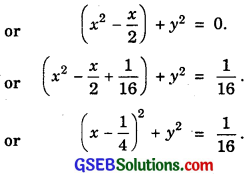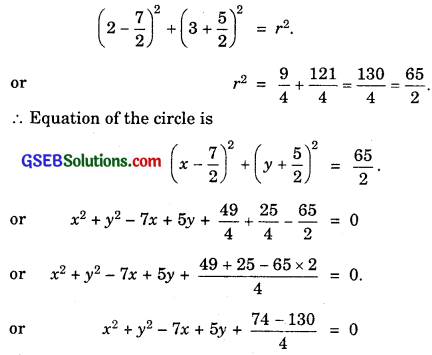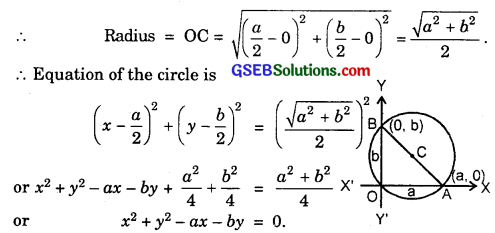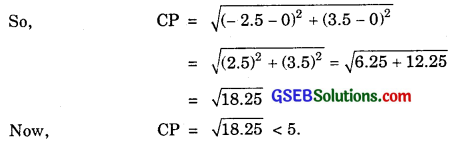# GSEB Solutions Class 11 Maths Chapter 11 Conic Sections Ex 11.1

Gujarat Board GSEB Textbook Solutions Class 11 Maths Chapter 11 Conic Sections Ex 11.1 Textbook Questions and Answers.

## Gujarat Board Textbook Solutions Class 11 Maths Chapter 11 Conic Sections Ex 11.1

In each of the following questions 1 to 5, find the equation of the circle with
1. Centre (0, 2) and radius 2.
2. Centre (- 2, 3) and radius 4.
3. Centre ($$\frac{1}{2}$$, $$\frac{1}{4}$$ and radius = $$\frac{1}{12}$$.
4. Centre (1, 1) and radius $$\sqrt{2}$$.
5. Centre (- a, – b) and radius $$\sqrt{a^{2}-b^{2}}$$.
Solutions to questions 1 to 5:
1. Centre of the circle (0, 2) and radius = 2.
∴ Equation of the circle is
(x – 0)2 + (y – 2)2 = 22.
or x2 + y2 – 4y = 0.

2. Centre of the circle is (- 2, 3) and radius is 4.
∴ Equation of the circle is
(x + 2)2 + (y – 3)2 = 42.
or x2 + y2 + 4x – 6y + 4 + 9 – 16 = 0 or x2 + y2 + 4x – 6y – 3 = 0.3. Centre of circle is ($$\frac{1}{2}$$, $$\frac{1}{4}$$ and radius is $$\frac{1}{12}$$.
∴ Equation of the circle is
(x – $$\frac{1}{2}$$)2 + (y – $$\frac{1}{4}$$)2 = $$\frac{1}{12^{2}}$$ = $$\frac{1}{144}$$.
or 144x2 + 144y2 – 144x – 72y + 45 = 1.
or 144x2 = 144y2 – 144x – 72y + 44 = 0.
or 36x2 + 36y2 – 36x – 18y + 11 = 0.

4. Centre of circle is (1, 1) and radius = $$\sqrt{2}$$
∴ Equation of circle is
(x – 1)2 + (y – 1)2 = ($$\sqrt{2}$$)2 = 2
or x2 + y2 – 2x – 2y + 2 = 2
or x2 + y2 – 2x – 2y = 0.

5. Centre of the circle is (- a, – b) and radius = $$\sqrt{a^{2}-b^{2}}$$
∴ Equation of circle is
(x + a)2 + (y + b)2 = a2 – b2.
or x2 + y2 + 2xa + 2yb + a2 + b2 = a2 – b2.
or x2 + y2 + 2ax + 2by + 2b2 = 0.In each of the following questions 6 to 9, find the centre and radius of the circle:
6. (x + 5)2 + (y – 3)2 = 36
7. x2 + y2 – 4x – 8y – 45 = 0
8. x2 + y2 – 8x + 10y – 12 = 0
9. 2x2 + 2y2 – x = 0.
Solutions to questions 6 to 9:
6. Comparing the equation of the circle
(x + 5)2 + (y – 3)2 = 36
with (x – h)2 + (y – k)2 = r2, we get
– h = 5 or h = – 5, k = 3, r2 = 36 or r = 6.
∴ Centre of the circle is (- 5, 3) and radius = 6.

7. Equation of the circle is
x2 + y2 – 4x – 8y = 45.
or (x2 – 4x) + (y2 – 8y) = 45
or (x2 – 4x + 4) + (y2 – 8y + 16) = 45 + 4 + 16 = 65.
⇒ (x – 2)2 + (y – 4)2 = 65
∴ Centre is (2, 4) and radius = $$\sqrt{65}$$.8. The equation of the circle is
x2 + y2 – 8x + 10y – 12
or (x2 – 8x) + (y2 + 10y) = 12.
or (x2 – 8x + 16) + (y2 + 10y + 25) = 12 + 16 + 25.
or (x – 4)2 + (y + 5)2 = 53.
∴ Centre is (4, – 5) and radius is $$\sqrt{53}$$.

9. Equation of the circle is
2x2 + 2y2 – x = 0
or x2 + y2 – $$\frac{x}{2}$$ = 0.∴ Centre is ($$\frac{1}{4}$$, 0) and radius = $$\frac{1}{4}$$.Question 10.
Find the equation of the circle passing through the points (4, 1) and (6, 5) and whose centre is on the line 4x + y = 16.
Solution:
Let the equation of the circle be
(x – h)2 + (y – k)2 = r2 ………………. (1)
The points (4, 1) and (6, 5) lies on it.
∴ (4 – h)2 + (1 – k)2 = r2
or h2 + k2 – 8h – 2k + 17 = r2 …………….. (2)
and (6 – h)2 + (5 – k)2 = r2
or h2 + k2 – 12h – 10k + 61 = r2 ………………….. (3)
The centre (h, k) lies on
4x + y = 16.
∴ 4h + k = 16 ………………….. (4)
Subtracting (3) from (2), we get
4h + 8k – 44
or h + 2k = 11 ……………………. (5)
Multiplying (5) by 4, we get
4h + 8k = 44.
Subtracting equation (4) from it, we get
7k = 44 – 16 = 28. ⇒ k = 4.
From (5), h + 8 = 11. ⇒ h = 3.
Putting h = 3, k = 4 in (2), we get
9 + 16 – 24 – 8 + 17 = r2
or 42 – 32 = r2
∴ r2 = 10.
∴ Equation of the circle is
(x – 3)2 + (y – 4)2 = 10
or x2 + y2 – 6x – 8y + 15 = 0.Question 11.
Find the equation of the circle passing through the points (2, 3) and (- 1, 1) and whose centre is on the line x – 3y – 11 = 0.
Solution:
Let the equation of the circle be
(x – h)2 + (y – k)2 = r2 ………………. (1)
The points (2, 3) and (- 1, 1) lies on it.
∴ (2 – h)2 + (3 – k)2 = r2
or h2 + k2 – 4h – 6k + 13 = r2 …………………… (2)
and (- 1 – h)2 + (1 – k)2 = r2 …………………. (3)
Centre (h, k) lies on x – 3y – 11 = 0.
∴ h – 3k – 11 = 0 …………….. (4)
Subtracting (2) from (3), we get
6h + 4k – 11 = 0 ………………. (5)
Multiplying (4) by 6, we get
6h – 18k – 66 = 0 …………………. (6)
Subtracting (6) from (5), we get
22k+ 55 = 0.
∴ k = – $$\frac{55}{22}$$ = – $$\frac{5}{2}$$.
From (4), h = 3k + 11 = – $$\frac{15}{2}$$ + 11 = $$\frac{7}{2}$$.
Putting the values of h and k in (2 – h)2 + (3 – k)2 = r2, we getor x2 + y2 – 7x + 5y – 14 = 0.Question 12.
Find the equation of the circle wi th radius 5, whose centre lies on x-axis and passes through the point (2, 3).
Solution:
Let the equation of the circle be
(x – h)2 + (y – k)2 = r2 ……………………. (1)
Here, r = 5, So, r2 = 25.
Centre lies onx-axis, i.e.,k = 0.
∴ Eq. (1) becomes
(x – h)2 + y2 = 25.
(2, 3) lies on it.
∴ (2 – h)2 + 9 = 25
or (2 – h)2 = 16
∴ 2 – h = ± 4
∴ h = – 2, 6
When h = – 2, equation of circle is
(x + 2)2 + y2 = 25
or x2 + y2 + 4x – 21 = 0.
When h = 6, equation of the circle is
(x – 6)2 + y2 = 25
x2 + y2 – 12x + 11 = 0.
Thus, required circles are
x2 + y2 + 4x – 21 = 0 and x2 + y2 – 12x + 11 = 0.Question 13.
Find the equation of the circle passing through (0, 0) and making intercepts a and b on the co-ordinate axes.
Solution:
a and b are the intercepts made by the circle on the co-ordinate axes at A and B. C, the mid-point of AB is the centre of the circle.
∴ Centre ($$\frac{a}{2}$$, $$\frac{b}{2}$$).Question 14.
Find the equation of a circle with centre (2, 2) and which passes through the point (4, 5).
Solution:
Centre of the circle C(2, 2).
P(4, 5) is a point on the circle.
∴ Radius = CP = $$\sqrt{(4-2)^{2}+(5-2)^{2}}$$
= $$\sqrt{4+9}$$ = $$\sqrt{13}$$.
∴ Equation of the circle is
(x – 2)2 + (y – 2)2 = 13.
or x2 + y2 – 4x – 4y = 5.Question 15.
Does the point (- 2.5, 3.5) lie inside, outside or on the circle x2 + y2 = 25?
Solution:
Centre C of the circle is (0, 0) and radius = 5.
The point is P(- 2.5, 3.5).